# Sound Speed in Gases

The speed of sound in an ideal gas is given by the relationship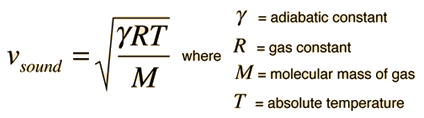Show

For air, the adiabatic constant γ = 1.4 and the average molecular mass for dry air is 28.95 gm/mol. This leads to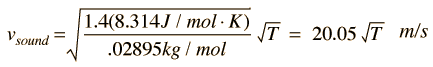Therefore for air at T = K = °C = °F ,

the speed of sound is vsound = m/s = ft/s = mi/hr.

It is interesting to compare this speed with the speed of molecules as a result of their thermal energy. For the specific example of dry air at 20°C, the speed of sound in air is 343 m/s, while the rms speed of air molecules is 502 m/s using a mean mass of air molecules of 29 amu.

For helium, γ = 5/3 and the molecular mass is .004 kg/mol, so its speed of sound at the same temperature is

vsound in helium = m/s = ft/s = mi/hr.

Doing this calculation for air at 0°C gives vsound = 331.39 m/s and at 1°C gives vsound = 332.00 m/s. This leads to a commonly used approximate formula for the sound speed in air: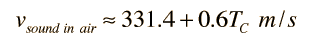For temperatures near room temperature, the speed of sound in air can be calculated from this convenient approximate relationship, but the more general relationship is needed for calculations in helium or other gases.

The calculation above was done for dry air, and moisture content in the air would be expected to increase the speed of sound slightly because the molecular weight of water vapor is 18 compared to 28.95 for dry air. A revised average molecular weight could be calculated based on the vapor pressure of water in the air. However, the assumption of an adiabatic constant of γ = 1.4 used in the calculation is based upon the diatomic molecules N2 and O2 and does not apply to water molecules. So the detailed modeling of the effect of water vapor on the speed of sound would have to settle on an appropriate value of γ to use.

 Table of sound speeds
Index

Traveling wave concepts

Sound propagation concepts

 HyperPhysics***** Sound R Nave
Go Back

# Sound Speed in an Ideal Gas

The speed of sound for a uniform medium is determined by its elastic property (bulk modulus) and its density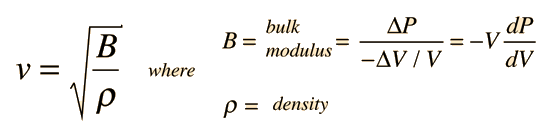When a sound travels through an ideal gas, the rapid compressions and expansions associated with the longitudinal wave can reasonably be expected to be adiabatic and therefore the pressure and volume obey the relationshipThe adiabatic assumption for sound waves just means that the compressions associated with the sound wave happen so quickly that there is no opportunity for heat transfer in or out of the volume of air. The bulk modulus can therefore be reformulated by making use of the adiabatic condition in the formso that the derivative of pressure P with respect to volume V can be taken.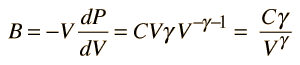Since the gas density is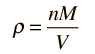the speed of sound can be expressed as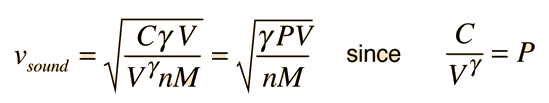Using the ideal gas law PV = nRT leads toThe conditions for this relationship are that the sound propagation process is adiabatic and that the gas obeys the ideal gas law.

 Calculation
Index

Traveling wave concepts

Sound propagation concepts

 HyperPhysics***** Sound R Nave
Go Back